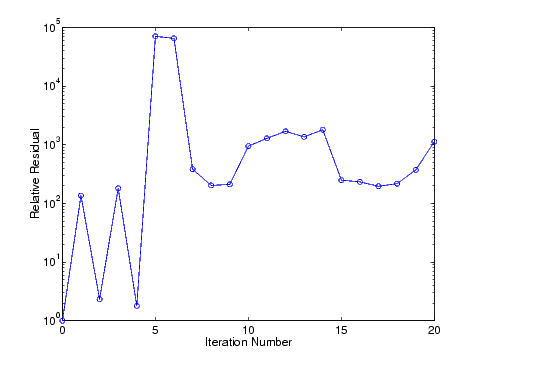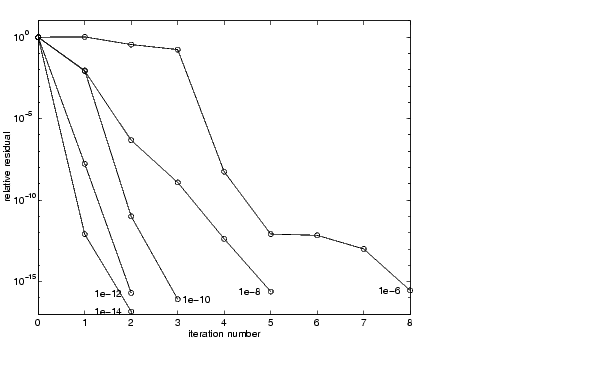MATLAB Function Referencebicg

Biconjugate gradients method

Syntax

• ```x = bicg(A,b)
bicg(A,b,tol)
bicg(A,b,tol,maxit)
bicg(A,b,tol,maxit,M)
bicg(A,b,tol,maxit,M1,M2)
bicg(A,b,tol,maxit,M1,M2,x0)
[x,flag] = bicg(A,b,...)
[x,flag,relres] = bicg(A,b,...)
[x,flag,relres,iter] = bicg(A,b,...)
[x,flag,relres,iter,resvec] = bicg(A,b,...)
```

Description

```x = bicg(A,b) ``` attempts to solve the system of linear equations `A*x = b` for `x`. The `n`-by-`n` coefficient matrix `A` must be square and should be large and sparse. The column vector `b` must have length `n`. `A` can be a function handle `afun` such that `afun(x,'notransp')` returns `A*x` and `afun(x,'transp')` returns `A'*x`. See Function Handles in the MATLAB Programming documentation for more information.

Parameterizing Functions Called by Function Functions, in the MATLAB Mathematics documentation, explains how to provide additional parameters to the function `afun`, as well as the preconditioner function `mfun` described below, if necessary.

If `bicg` converges, it displays a message to that effect. If `bicg` fails to converge after the maximum number of iterations or halts for any reason, it prints a warning message that includes the relative residual `norm(b-A*x)/norm(b)` and the iteration number at which the method stopped or failed.

```bicg(A,b,tol) ``` specifies the tolerance of the method. If `tol` is `[]`, then `bicg` uses the default, `1e-6`.

```bicg(A,b,tol,maxit) ``` specifies the maximum number of iterations. If `maxit` is `[]`, then `bicg` uses the default, `min(n,20)`.

```bicg(A,b,tol,maxit,M) and bicg(A,b,tol,maxit,M1,M2) ``` use the preconditioner `M` or `M = M1*M2` and effectively solve the system `inv(M)*A*x = inv(M)*b` for `x`. If `M` is `[]` then `bicg` applies no preconditioner. `M` can be a function handle `mfun` such that `mfun(x,'notransp')` returns `M\x `and `mfun(x,'transp')` returns `M'\x`.

```bicg(A,b,tol,maxit,M1,M2,x0) ``` specifies the initial guess. If `x0` is `[]`, then `bicg` uses the default, an all-zero vector.

```[x,flag] = bicg(A,b,...) ``` also returns a convergence flag.

 Flag Convergence `0` `bicg `converged to the desired tolerance `tol` within `maxit `iterations. `1` `bicg `iterated `maxit` times but did not converge. `2` Preconditioner `M` was ill-conditioned. `3` `bicg` stagnated. (Two consecutive iterates were the same.) `4` One of the scalar quantities calculated during `bicg` became too small or too large to continue computing.

Whenever `flag` is not `0`, the solution `x` returned is that with minimal norm residual computed over all the iterations. No messages are displayed if the `flag` output is specified.

```[x,flag,relres] = bicg(A,b,...) ``` also returns the relative residual `norm(b-A*x)/norm(b)`. If `flag` is `0`, `relres <= tol`.

```[x,flag,relres,iter] = bicg(A,b,...) ``` also returns the iteration number at which `x` was computed, where `0 <= iter <= maxit`.

```[x,flag,relres,iter,resvec] = bicg(A,b,...) ``` also returns a vector of the residual norms at each iteration including `norm(b-A*x0)`.

Examples

Example 1.

• ```n = 100;
on = ones(n,1);
A = spdiags([-2*on 4*on -on],-1:1,n,n);
b = sum(A,2);
tol = 1e-8;
maxit = 15;
M1 = spdiags([on/(-2) on],-1:0,n,n);
M2 = spdiags([4*on -on],0:1,n,n);

x = bicg(A,b,tol,maxit,M1,M2);
```

displays this message

• ```bicg converged at iteration 9 to a solution with relative
residual 5.3e-009
```

Example 2.

This example replaces the matrix `A` in Example 1 with a handle to a matrix-vector product function `afun`. The example is contained in an M-file `run_bicg` that

• Calls `bicg` with the function handle `@afun` as its first argument.
• Contains `afun` as a nested function, so that all variables in `run_bicg` are available to `afun`.

The following shows the code for `run_bicg`:

• ```function x1 = run_bicg
n = 100;
on = ones(n,1);
A = spdiags([-2*on 4*on -on],-1:1,n,n);
b = sum(A,2);
tol = 1e-8;
maxit = 15;
M1 = spdiags([on/(-2) on],-1:0,n,n);
M2 = spdiags([4*on -on],0:1,n,n);
x1 = bicg(@afun,b,tol,maxit,M1,M2);

function y = afun(x,transp_flag)
if strcmp(transp_flag,'transp')      % y = A'*x
y = 4 * x;
y(1:n-1) = y(1:n-1) - 2 * x(2:n);
y(2:n) = y(2:n) - x(1:n-1);
elseif strcmp(transp_flag,'notransp') % y = A*x
y = 4 * x;
y(2:n) = y(2:n) - 2 * x(1:n-1);
y(1:n-1) = y(1:n-1) - x(2:n);
end
end
end
```

When you enter

• ```x1=run_bicg;
```

MATLAB displays the message

• ```bicg converged at iteration 9 to a solution with relative residual
5.3e-009
```

Example 3. This example demonstrates the use of a preconditioner. Start with `A = west0479`, a real 479-by-479 sparse matrix, and define `b` so that the true solution is a vector of all ones.

• ```load west0479;
A = west0479;
b = sum(A,2);
```

You can accurately solve `A*x = b` using backslash since `A `is not so large.

• ```x = A \ b;
norm(b-A*x) / norm(b)

ans =
8.3154e-017
```

Now try to solve` A*x = b` with `bicg`.

• ```[x,flag,relres,iter,resvec] = bicg(A,b)

flag =
1
relres =
1
iter =
0
```

The value of `flag` indicates that `bicg` iterated the default 20 times without converging. The value of `iter` shows that the method behaved so badly that the initial all-zero guess was better than all the subsequent iterates. The value of `relres` supports this: `relres = norm(b-A*x)/norm(b`) = `norm(b)/norm(b)` = `1`. You can confirm that the unpreconditioned method oscillates rather wildly by plotting the relative residuals at each iteration.

• ```s```emilogy(0:20,resvec/norm(b),'-o')
```xlabel('Iteration Number')
ylabel('Relative Residual')```

Now, try an incomplete LU factorization with a drop tolerance of `1e-5` for the preconditioner.

• ```[L1,U1] = luinc(A,1e-5);
Warning: Incomplete upper triangular factor has 1 zero diagonal.
It cannot be used as a preconditioner for an iterative
method.

nnz(A), nnz(L1), nnz(U1)

ans =
1887
ans =
5562
ans =
4320
```

The zero on the main diagonal of the upper triangular `U1` indicates that `U1` is singular. If you try to use it as a preconditioner,

• ```[x,flag,relres,iter,resvec] = bicg(A,b,1e-6,20,L1,U1)

flag =
2
relres =
1
iter =
0
resvec =
7.0557e+005
```

the method fails in the very first iteration when it tries to solve a system of equations involving the singular `U1` using backslash. `bicg` is forced to return the initial estimate since no other iterates were produced.

Try again with a slightly less sparse preconditioner.

• ```[L2,U2] = luinc(A,1e-6);

nnz(L2), nnz(U2)

ans =
6231
ans =
4559
```

This time `U2` is nonsingular and may be an appropriate preconditioner.

• ```[x,flag,relres,iter,resvec] = bicg(A,b,1e-15,10,L2,U2)

flag =
0
relres =
2.8664e-016
iter =
8
```

and `bicg` converges to within the desired tolerance at iteration number 8. Decreasing the value of the drop tolerance increases the fill-in of the incomplete factors but also increases the accuracy of the approximation to the original matrix. Thus, the preconditioned system becomes closer to `inv(U)*inv(L)*L*U*x = inv(U)*inv(L)*b`, where `L` and `U` are the true LU factors, and closer to being solved within a single iteration.

The next graph shows the progress of `bicg` using six different incomplete LU factors as preconditioners. Each line in the graph is labeled with the drop tolerance of the preconditioner used in `bicg`.See Also

`bicgstab`, `cgs`, `gmres`, `lsqr`, `luinc`, `minres`, `pcg`, `qmr`, `symmlq`

`function_handle` (`@`), `mldivide` (`\`)

References

  Barrett, R., M. Berry, T. F. Chan, et al., Templates for the Solution of Linear Systems: Building Blocks for Iterative Methods, SIAM, Philadelphia, 1994.

© 1994-2005 The MathWorks, Inc.